# MS Excel Must know Upper lower len Pivot function

## MS Excel Most useful functions and tricks of MS Excel:

MS Excel Must know Upper lower len Pivot function

## 1) Use of Len:

it is used to determine and get the length of characters in a cell of MS Excel. The formula is very simple. Let us assume that we want to determine the length of characters in cell A1. In this case the formula will be:
=len(A1)

Illustration: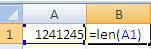MS Excel cell length and len function-1

And the result of Len formula will be showing us the number of characters in a cell of MS Excel as depicted below: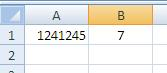MS Excel cell length and len function-2

## 2) How to convert text in MS excel cell from lowercase to upper case?

This is quite easy to do with “UPPER” function of MS excel which converts small text to upper case text. An example is shown below:
Illustration: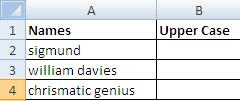MS Excel Text Upper Case function-1

Let us say that we want to change the case of text in below table:

Formula for upper case conversion: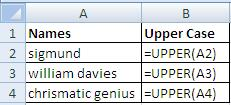MS Excel Convert text to upper case-2

Result of this formula for Converting to Upper case text: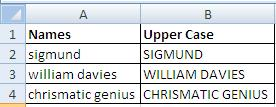MS Excel Change text case to upper-3

## 3) Converting Excel text from UPPER case to Lower Case:

Let us assume that we wish to convert text in the below table from upper case to lower case:MS Excel change text case to lower-1

Applying formula for transforming Upper case to lower case: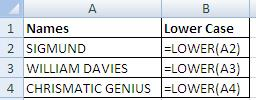Change text to lower case MS excel-2

Result of MS Excel converting upper case text in cell to lower case text: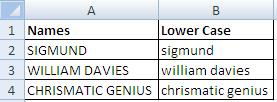Convert text to lower case MS excel

## 4) Converting MS Excel cell text to proper case

It means that every first character shall be capital while other letters shall be in small font:Convert text to proper case MS Excel-1

Illustration of Formula for using MS Excel Proper Function to convert text from any format to proper format: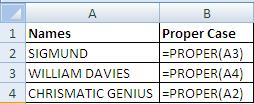Change text to proper case MS excel-3

Result of Proper function using MS excel: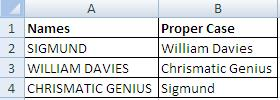MS excel convert text to proper case-3

## 5) Quickly generating Excel Data summary using Pivot Table: Let us say we have the below table in excel and want to have a quick summary of different items city wise. The easiest way is to insert a Pivot Table.

Select the entire table by hitting Ctrl+A and then click insert:MS excel quick use of PivotTable-1

Hit PivotTable Icon on the extreme left. Click OK.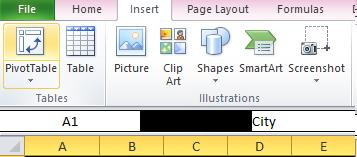PivotTable for summary MS Excel-2

Check the required boxes and there you go: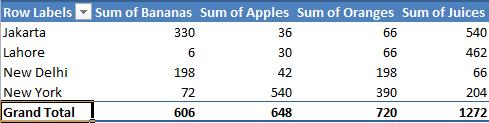MS Excel Pivot Table-3

The above summary is auto generated without any errors and omissions. A real smooth way for doing sum and calculation is using PivotTable, the best practice in